# Hidden Markov Models Decoding – The Viterbi Algorithm

## Decoding

Given as input an HMM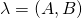and a sequence of observations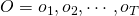, determine the most probable sequence of states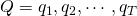that produced those observations.

## Viterbi Algorithm

The Viterbi Algorithm is similar to another dynamic programming problem – the minimum edit distance algorithm. The Viterbi algorithm is identical to the Forward algorithm except that it takes the max over the previous path probabilities whereas the forward algorithm takes the sum.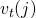represents the probability that the HMM is in state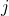after seeing the firstobservations and passing through the most probable state sequence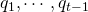, given the HMM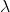. The most probable path is represented by taking the maximum over all possible previous state sequences.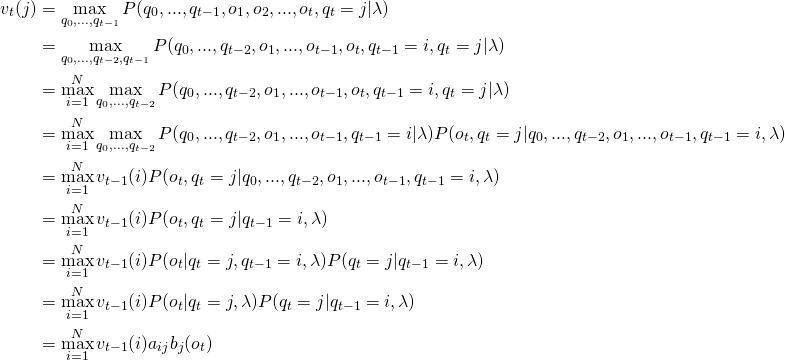where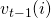is the previous Viterbi path probability from the previous time step.

## Backpointers

The Viterbi algorithm also keeps track of backpointers to produce the most likely state sequence. At the end, backtracking via these backpointers results in the best path to the beginning.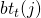stores the state at time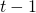that maximizes the probability that system was in stateat time(given the observed sequence).

## Visualization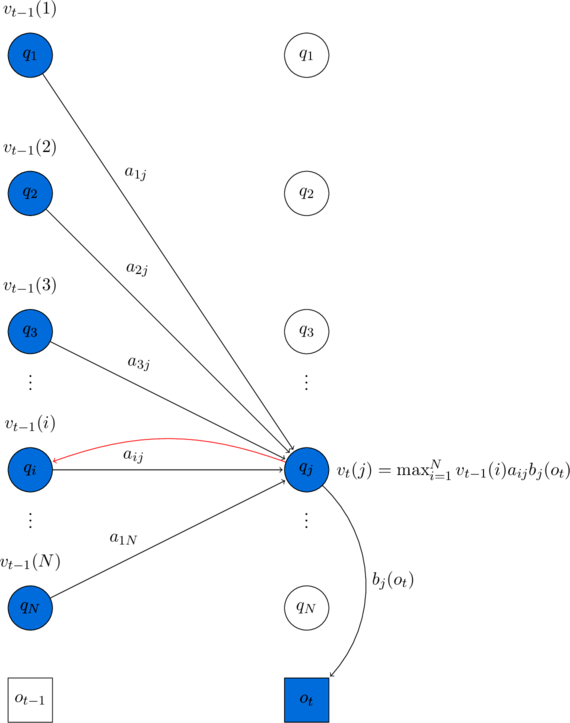Visualizing the computation of a single elementin the trellis by finding the maximum among all the paths from, weighted by their transition probabilities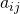, and multiplying by the observation probability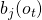. Hidden states are in circles, observations in squares. Shaded nodes are included in the probability computation for. As each path is extended to a new state to account for the next observation, a backpointer (shown in red) keeps track of the best path that led to this state.

## Initialization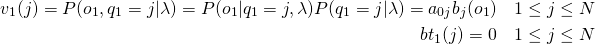## Recursion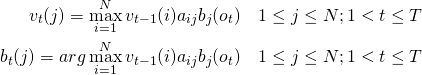## Termination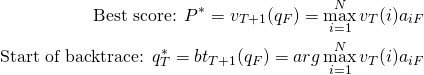## Backtrack Path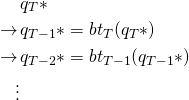## Time Complexity

Initialization step runs in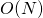. Recursion step is run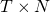times and the summation is over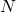. Thus, the recursion step runs in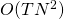. Termination step runs in. The forward algorithm thus reduces the time complexity to.